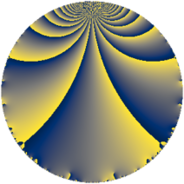Properties

 Label 126.3.qLevel $126$ Weight $3$ Character orbit 126.q Rep. character $\chi_{126}(29,\cdot)$ Character field $\Q(\zeta_{6})$ Dimension $24$ Newform subspaces $1$ Sturm bound $72$ Trace bound $0$

Related objects

Defining parameters

 Level: $$N$$ $$=$$ $$126 = 2 \cdot 3^{2} \cdot 7$$ Weight: $$k$$ $$=$$ $$3$$ Character orbit: $$[\chi]$$ $$=$$ 126.q (of order $$6$$ and degree $$2$$) Character conductor: $$\operatorname{cond}(\chi)$$ $$=$$ $$9$$ Character field: $$\Q(\zeta_{6})$$ Newform subspaces: $$1$$ Sturm bound: $$72$$ Trace bound: $$0$$

Dimensions

The following table gives the dimensions of various subspaces of $$M_{3}(126, [\chi])$$.

Total New Old
Modular forms 104 24 80
Cusp forms 88 24 64
Eisenstein series 16 0 16

Trace form

 $$24 q + 24 q^{4} + 36 q^{5} + 8 q^{6} - 32 q^{9} + O(q^{10})$$ $$24 q + 24 q^{4} + 36 q^{5} + 8 q^{6} - 32 q^{9} - 24 q^{12} - 44 q^{15} - 48 q^{16} + 48 q^{18} + 24 q^{19} + 72 q^{20} + 28 q^{21} + 24 q^{22} - 72 q^{23} - 16 q^{24} + 72 q^{25} - 108 q^{29} - 56 q^{30} - 60 q^{31} + 104 q^{33} - 48 q^{34} - 80 q^{36} - 168 q^{37} + 144 q^{38} + 64 q^{39} + 108 q^{41} + 60 q^{43} + 116 q^{45} - 324 q^{47} - 48 q^{48} - 84 q^{49} + 144 q^{50} - 268 q^{51} - 208 q^{54} + 264 q^{55} + 60 q^{57} - 432 q^{59} - 32 q^{60} - 192 q^{64} - 180 q^{65} - 112 q^{66} + 72 q^{67} + 72 q^{68} + 536 q^{69} + 96 q^{72} + 24 q^{73} + 288 q^{74} + 76 q^{75} + 24 q^{76} - 64 q^{78} + 12 q^{79} + 544 q^{81} - 288 q^{82} + 756 q^{83} + 112 q^{84} - 156 q^{85} + 360 q^{86} + 280 q^{87} - 48 q^{88} + 80 q^{90} - 168 q^{91} - 144 q^{92} + 436 q^{93} - 936 q^{95} - 64 q^{96} + 48 q^{97} - 440 q^{99} + O(q^{100})$$

Decomposition of $$S_{3}^{\mathrm{new}}(126, [\chi])$$ into newform subspaces

Label Dim. $$A$$ Field CM Traces $q$-expansion
$a_{2}$ $a_{3}$ $a_{5}$ $a_{7}$
126.3.q.a $24$ $3.433$ None $$0$$ $$0$$ $$36$$ $$0$$

Decomposition of $$S_{3}^{\mathrm{old}}(126, [\chi])$$ into lower level spaces

$$S_{3}^{\mathrm{old}}(126, [\chi]) \cong$$ $$S_{3}^{\mathrm{new}}(9, [\chi])$$$$^{\oplus 4}$$$$\oplus$$$$S_{3}^{\mathrm{new}}(18, [\chi])$$$$^{\oplus 2}$$$$\oplus$$$$S_{3}^{\mathrm{new}}(63, [\chi])$$$$^{\oplus 2}$$